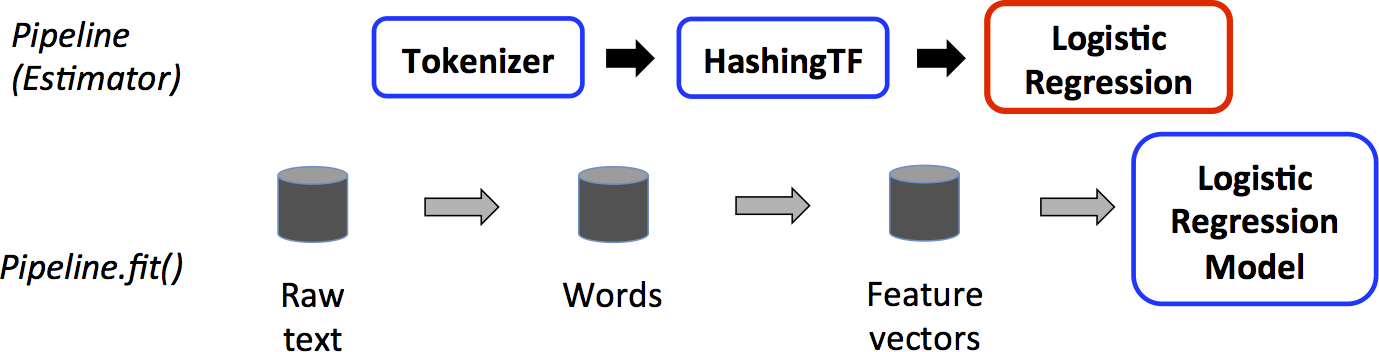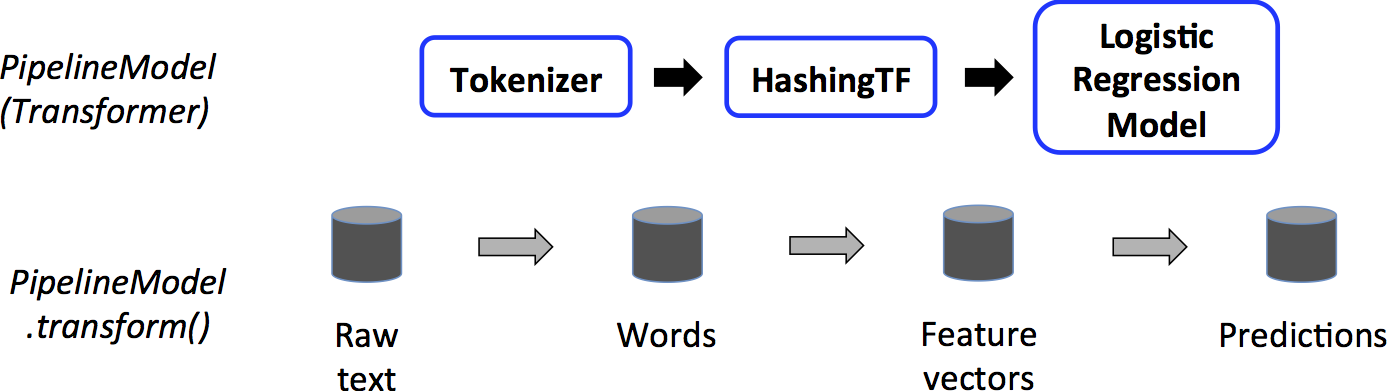##### 页面树结构

2017-07-25 Apache Spark 2.2.0 官方文档中文版发布 : http://spark.apachecn.org/docs/cn/2.2.0/

MachineLearning 优酷地址 : http://i.youku.com/apachecn

# Pipelines的主要概念

MLlib 将机器学习算法的API标准化，以便将多种算法更容易地组合成单个 Pipeline （管道）或者工作流。本节介绍Pipelines API 的关键概念,其中 Pipeline（管道）的概念主要是受到 scikit-learn 项目的启发.

• DataFrame（数据模型）：ML API 将从Spark SQL查出来的 DataFrame 作为 ML 的数据集,数据集支持许多数据类型。例如,一个 DataFrame 可以有不同的列储存 text（文本）、feature（特征向量）、true labels（标注）、predictions（预测结果）等机器学习数据类型.
• Transformer（转换器）：使用 Transformer 将一个 DataFrame 转换成另一个 DataFrame 的算法，例如，一个 ML Model 一个 Transformer,它将带有特征的 DataFrame 转换成带有预测结果的 DataFrame.
• Estimator（模型学习器）：Estimator 是一个适配 DataFrame 来生成 Transformer（转换器）的算法.例如,一个学习算法就是一个 Estimator 训练 DataFrame 并产生一个模型的过程.
• Pipeline（管道）：Pipeline 将多个 `Transformer`和 `Estimator`s 绑在一起形成一个工作流.
• Parameter（参数）：所有的 `Transformer`和 `Estimator`都已经使用标准的 API 来指定参数.

## DataFrame

DataFrame 支持许多基本的结构化的数据，参考Spark SQL datatype reference上的一系列支持的类型。另外除了 Spark SQL guide 列举的类型,DataFrame 还支持使用 ML Vector 类型.

DataFrame 可以用标准的 RDD 显式或者非显式创建。请参考下面的例子，或者前往 Spark SQL programming guide 查看例子

DataFrame 中的列是有名称的.下面的代码示例会使用名称如 "文本","特征"和"标签".

## Pipelines 组件

### Transformers（转换器）

转换器是特征变换和机器学习模型的抽象。转换器必须实现transform方法，这个方法将一个 DataFrame 转换成另一个 DataFrame，通常是附加一个或者多个列。比如：

• 一个特征变换器是输入一个 DataFrame，读取一个列（比如：text），将其映射成一个新列（比如，特征向量），然后输出一个新的 DataFrame 并包含这个映射的列.
• 一个机器学习模型是输入一个 DataFrame，读取包含特征向量的列,预测每个特征向量的标签,并输出一个新的 DataFrame ，并附加预测标签作为一列.

### Estimators（模型学习器）

Estimators 模型学习器是拟合和训练数据的机器学习算法或者其他算法的抽象。技术上来说, Estimator（模型学习器）实现 fit() 方法，这个方法输入一个 DataFrame 并产生一个 Model 即一个 Transformer（转换器）。举个例子，一个机器学习算法是一个 Estimator 模型学习器,比如这个算法是 LogisticRegression（逻辑回归），调用 fit() 方法训练出一个 LogisticRegressionModel,这是一个 Model,因此也是一个 Transformer（转换器）.

### Pipeline组件的参数

Transformer.transform() 和 Estimator.fit() 都是无状态。以后,可以通过替换概念来支持有状态算法.

## Pipeline

• 把每个文档的文本分割成单词.
• 将这些单词转换成一个数值型特征向量.
• 使用特征向量和标签学习一个预测模型.

MLlib 代表一个流水线,就是一个 Pipeline（管道）Pipeline（管道） 包含了一系列有特定顺序的管道步骤（`Transformer`s（转换器） 和 `Estimator`s（模型学习器）。我们将使用这个简单的工作流作为本节的运行示例.

### 如何工作Pipeline 和 PipelineModel 有助于确保了训练集和测试集经过相同的处理步骤.

### 细节

DAG（有向无环图）`Pipeline`s：管道的步骤被定义为一个有序的数组。上面的例子是一个线性的管道即 `Pipeline`s每个步骤使用的数据都产生于前一个步骤。只要数据流图能构成有向无环图，非线性 Pipelines 也可以创建出来。图当前是通过输每个步骤的输入输出列名来隐含指定的（通常指定为参数）.如果 Pipeline 构成了一个 DAG,则按照拓扑顺序指定阶段.

Runtime checking运行时检查）：由于管道可以对具有不同类型的 DataFrames 进行操作,因此不能使用编译时类型检查。`Pipeline``PipelineModel`在真正运行 Pipeline 之前进行运行时检查。这个类型检查通过 DataFrame schema（描述了 DataFrame 列的数据类型）来完成的.

Unique Pipeline stages（唯一性管道阶段）：一个管道的阶段必须是一个唯一实例，比如，相同的实例 myHashingTF不能插入到 Pipeline 两次，因为管道的阶段的 ID 必须是唯一的。但是，不同的实例 myHashingTF1 myHashingTF2（都是 HashingTF 类型）可以放进相同的 Pipeline,只要通过不同的 ID 创建不同的实例.

## 参数

MLib 的 `Estimator`s（模型学习器）和 `Transformer`s转换器）使用统一的 API 来指定参数.

Param 是具有自包含定义的参数，ParamMap 是一组（参数,值）对.

1. 给实例设置参数,例如,如果 lr 是一个 LogisticRegression实例,调用 `lr.setMaxIter(10)` 使 `lr.fit()` 最多10次迭代。这个 API 类似于 spark.mlib 包中的 API.
2. 传递一个 ParamMap  `fit()` 函数和 `transform() `函数。ParamMap 的任意参数将会覆盖前面调用实例通过 setter 方法指定的参数.

# 示例代码

## 例子：Estimator（模型学习器）,Transformer（转换器），Param（参数）

Scala
```import org.apache.spark.ml.classification.LogisticRegression
import org.apache.spark.ml.linalg.{Vector, Vectors}
import org.apache.spark.ml.param.ParamMap
import org.apache.spark.sql.Row

// Prepare training data from a list of (label, features) tuples.
val training = spark.createDataFrame(Seq(
(1.0, Vectors.dense(0.0, 1.1, 0.1)),
(0.0, Vectors.dense(2.0, 1.0, -1.0)),
(0.0, Vectors.dense(2.0, 1.3, 1.0)),
(1.0, Vectors.dense(0.0, 1.2, -0.5))
)).toDF("label", "features")

// Create a LogisticRegression instance. This instance is an Estimator.
val lr = new LogisticRegression()
// Print out the parameters, documentation, and any default values.
println("LogisticRegression parameters:\n" + lr.explainParams() + "\n")

// We may set parameters using setter methods.
lr.setMaxIter(10)
.setRegParam(0.01)

// Learn a LogisticRegression model. This uses the parameters stored in lr.
val model1 = lr.fit(training)
// Since model1 is a Model (i.e., a Transformer produced by an Estimator),
// we can view the parameters it used during fit().
// This prints the parameter (name: value) pairs, where names are unique IDs for this
// LogisticRegression instance.
println("Model 1 was fit using parameters: " + model1.parent.extractParamMap)

// We may alternatively specify parameters using a ParamMap,
// which supports several methods for specifying parameters.
val paramMap = ParamMap(lr.maxIter -> 20)
.put(lr.maxIter, 30)  // Specify 1 Param. This overwrites the original maxIter.
.put(lr.regParam -> 0.1, lr.threshold -> 0.55)  // Specify multiple Params.

// One can also combine ParamMaps.
val paramMap2 = ParamMap(lr.probabilityCol -> "myProbability")  // Change output column name.
val paramMapCombined = paramMap ++ paramMap2

// Now learn a new model using the paramMapCombined parameters.
// paramMapCombined overrides all parameters set earlier via lr.set* methods.
val model2 = lr.fit(training, paramMapCombined)
println("Model 2 was fit using parameters: " + model2.parent.extractParamMap)

// Prepare test data.
val test = spark.createDataFrame(Seq(
(1.0, Vectors.dense(-1.0, 1.5, 1.3)),
(0.0, Vectors.dense(3.0, 2.0, -0.1)),
(1.0, Vectors.dense(0.0, 2.2, -1.5))
)).toDF("label", "features")

// Make predictions on test data using the Transformer.transform() method.
// LogisticRegression.transform will only use the 'features' column.
// Note that model2.transform() outputs a 'myProbability' column instead of the usual
// 'probability' column since we renamed the lr.probabilityCol parameter previously.
model2.transform(test)
.select("features", "label", "myProbability", "prediction")
.collect()
.foreach { case Row(features: Vector, label: Double, prob: Vector, prediction: Double) =>
println(s"(\$features, \$label) -> prob=\$prob, prediction=\$prediction")
}```

参考:

[`Estimator` Scala docs](api/scala/index.html#org.apache.spark.ml.Estimator)

[`Transformer` Scala docs](api/scala/index.html#org.apache.spark.ml.Transformer) 和

[`Params` Scala docs](api/scala/index.html#org.apache.spark.ml.param.Params)了解API的详细信息。

## 示例：Pipeline

Scala
```import org.apache.spark.ml.{Pipeline, PipelineModel}
import org.apache.spark.ml.classification.LogisticRegression
import org.apache.spark.ml.feature.{HashingTF, Tokenizer}
import org.apache.spark.ml.linalg.Vector
import org.apache.spark.sql.Row

// Prepare training documents from a list of (id, text, label) tuples.
val training = spark.createDataFrame(Seq(
(0L, "a b c d e spark", 1.0),
(1L, "b d", 0.0),
(2L, "spark f g h", 1.0),
(3L, "hadoop mapreduce", 0.0)
)).toDF("id", "text", "label")

// Configure an ML pipeline, which consists of three stages: tokenizer, hashingTF, and lr.
val tokenizer = new Tokenizer()
.setInputCol("text")
.setOutputCol("words")
val hashingTF = new HashingTF()
.setNumFeatures(1000)
.setInputCol(tokenizer.getOutputCol)
.setOutputCol("features")
val lr = new LogisticRegression()
.setMaxIter(10)
.setRegParam(0.001)
val pipeline = new Pipeline()
.setStages(Array(tokenizer, hashingTF, lr))

// Fit the pipeline to training documents.
val model = pipeline.fit(training)

// Now we can optionally save the fitted pipeline to disk
model.write.overwrite().save("/tmp/spark-logistic-regression-model")

// We can also save this unfit pipeline to disk
pipeline.write.overwrite().save("/tmp/unfit-lr-model")

// And load it back in during production
val sameModel = PipelineModel.load("/tmp/spark-logistic-regression-model")

// Prepare test documents, which are unlabeled (id, text) tuples.
val test = spark.createDataFrame(Seq(
(4L, "spark i j k"),
(5L, "l m n"),
(6L, "spark hadoop spark"),
)).toDF("id", "text")

// Make predictions on test documents.
model.transform(test)
.select("id", "text", "probability", "prediction")
.collect()
.foreach { case Row(id: Long, text: String, prob: Vector, prediction: Double) =>
println(s"(\$id, \$text) --> prob=\$prob, prediction=\$prediction")
}```

• 无标签